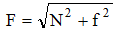Ex﻿

2. Force & Laws Of Motion

Force

The external agent which tends to set a body in motion or which changes the speed and direction of motion of a body or which can change the shape of a body is called force. SI unit of force is newton.
Types of forces :
(a) Non Contact Forces :
These are the forces in which contact between two objects is not necessary. Gravitational force between two bodies and electrostatic force between two charges are two examples of field forces.
(b) Contact Forces :
Two bodies in contact exert equal and opposite forces on each other. If the contact is frictionless the contact force is perpendicular to the common surface and known as normal reaction.If, however, the objects are in rough contact and move (or have a tendency to move) relative to each other without losing contact then frictional force arise which oppose such motion. Again each object exerts a frictional force on the other and the two forces are equal and opposite. This force is perpendicular to normal reaction. Thus, the contact force (F) between two objects is made up of two forces.
(i) Normal reaction (N) (ii) Force of friction (f)and since these two forces are mutually perpendicular.If you want to give information about online courses to other students, then share it with more and more on Facebook, Twitter, Google Plus. The more the shares will be, the more students will benefit. The share buttons are given below for your convenience.
×

NTSE Physics (Class X)

• Electricity
• Magnetic Effect of Curent
• Light Reflection
• Light Refraction
• Human Eye & Colourful World
• Source of Energy
• Motion

NTSE Physics (Class IX)

• Force & laws of motion
• Work and Energy
• Gravitation
• Sound

SHOW CHAPTERS